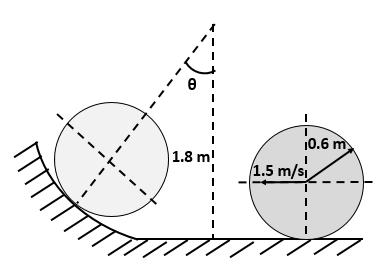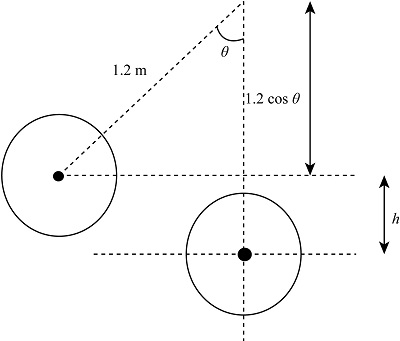# As the homogenous cylinder of mass ''m'' enter the cylindrical surface, the velocity of its...

## Question:

As the homogenous cylinder of mass m enter the cylindrical surface, the velocity of its center is 1.5 m/s. Determine the angle {eq}\theta {/eq} at which the cylinder will come to a momentary stop. Assume that the cylinder rolls without slipping.## Conservation of energy:

It states that for a body in linear or rotational motion, the kinetic energy gained by the body is equal to the potential energy lost by the body during its motion.

## Answer and Explanation:

Given Data

• The velocity of centre of cylinder is: {eq}v = 1.5\;{\rm{m/}}{{\rm{s}}^{\rm{}}} {/eq}

The figure below represents the height gained by the cylinder.The mass moment of inertia of the cylinder is,

{eq}I = \dfrac{{m{r^2}}}{2} {/eq}

The energy equation for the cylinder is,

{eq}K.{E_{lost}} = P.{E_{gain}} ...... (I) {/eq}

Here, the loss in kinetic energy of the cylinder is {eq}K.{E_{loss}} {/eq} and the gain in the potential energy of the cylinder is {eq}P.{E_{gain}}. {/eq}

The height gained by the cylinder is,

{eq}\begin{align*}{l} h &= 1.2 - 1.2\cos \theta \\ & = 1.2\left( {1 - \cos \theta } \right) \end{align*} {/eq}

The gain in potential energy is,

{eq}P.{E_{gain}} = mg\left( {1.2\left( {1 - \cos \theta } \right)} \right) {/eq}

The loss in kinetic energy is,

{eq}K.{E_{lost}} = \dfrac{{m{v^2}}}{2} + \dfrac{{I{\omega ^2}}}{2} {/eq}

Substitute the values in above equation.

{eq}\begin{align*} K.{E_{lost}}& = \dfrac{{m{v^2}}}{2} + \dfrac{{m{r^2}{\omega ^2}}}{4}\\ & = \dfrac{{m{v^2}}}{2} + \dfrac{{m{v^2}}}{4}\\ & = \dfrac{{3m{v^2}}}{4} \end{align*} {/eq}

Substitute the values in equation (I).

{eq}\begin{align*} mg\left( {1.2\left( {1 - \cos \theta } \right)} \right)& = \dfrac{{3m{v^2}}}{4}\\ g\left( {1.2\left( {1 - \cos \theta } \right)} \right)& = \dfrac{{3{v^2}}}{4} \end{align*} {/eq}

Substitute the values in above equation.

{eq}\begin{align*} 9.81\left( {1.2\left( {1 - \cos \theta } \right)} \right)& = \dfrac{{3{{\left( {1.5} \right)}^2}}}{4}\\ 1 - \cos \theta & = 0.1433\\ \theta & = 31.05^\circ \end{align*} {/eq}

Thus, the angle at which cylinder stops is {eq}31.05^\circ {/eq}